# Subtraction

Subtraction is all about taking one number away from another. You might use the words "take away" when you talk about subtraction.

For example, if we start out with $8$ carrots: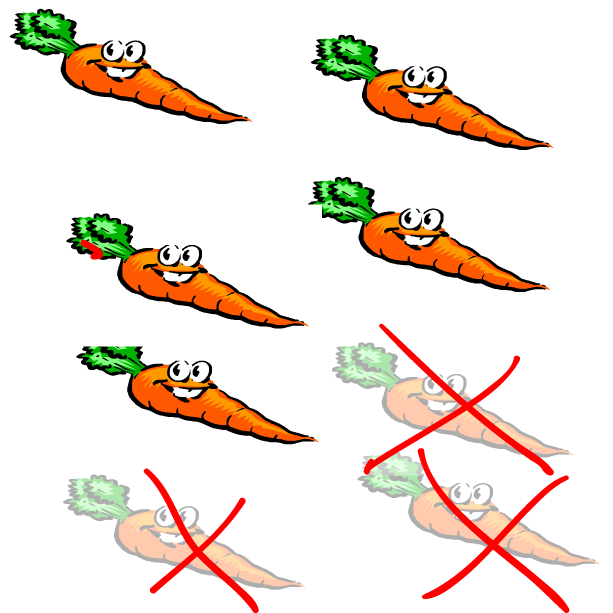and take $3$ away, we end up with $5$ carrots. We can write this, using the symbol $-$ (minus) for subtraction as:

$8 - 3 = 5$

Let's try another example. Suppose you have $6$ pears and take $2$ away: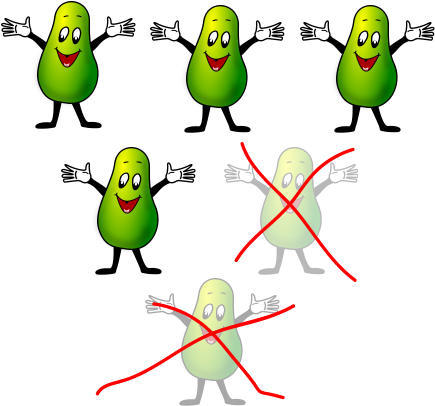Then you have $4$ pears left. We can write this as:
$6 - 2 = 4$

### Get Plenty of Practice

The only way to get good at subtraction is by doing plenty of it. So, practise whenever you can. You can practise subtracting with everyday objects like your peas at dinner. Each time you eat one, think "I am subtracting one pea, so I have seventy-seven (or however many there are) left. $78 - 1 = 77$."

Some other things you can practise subtraction with are sea shells and coins. In fact, you can practise your subtraction using any object that you have more than one of.

### Using Subtraction Tables

One technique for doing subtraction is to use a subtraction grid like the one below to look up the answers. If you do this often enough, you'll find that you have started to memorise the answers. It's a good idea to remember all the results of subtracting the numbers from $0$ to $10$ from each other as this will help you with harder subtraction sums later.

Let's use the grid to work out what $7 - 4$ is: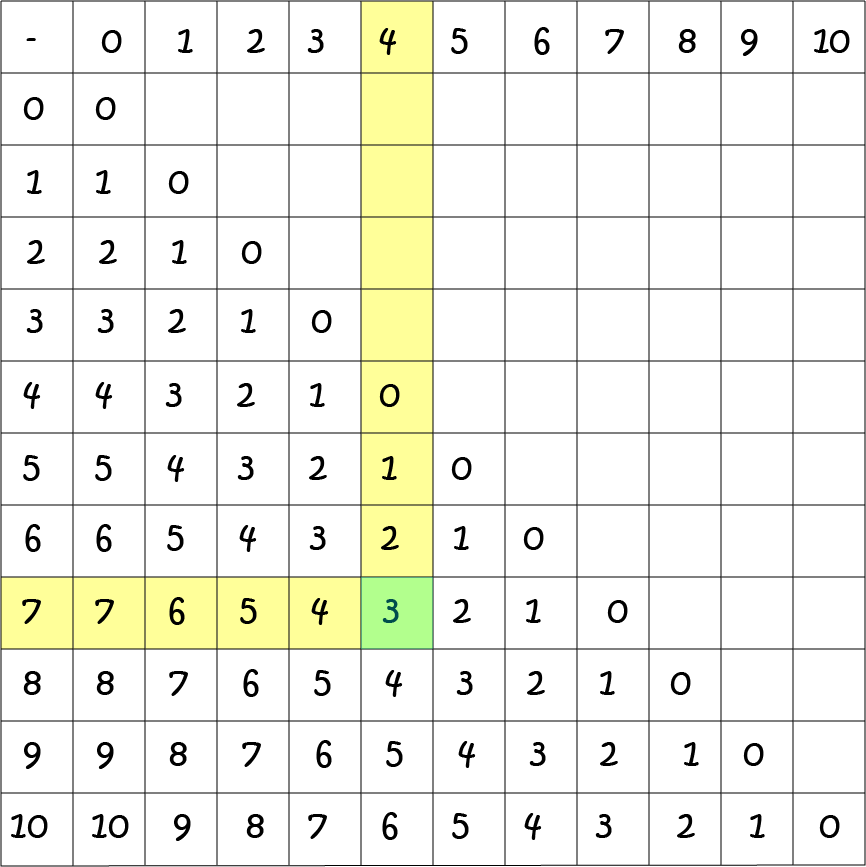1. First, find the row starting with "7"
2. Next, work your way along that row until you reach the column that is labelled by "4" at the top.
3. The number in that square is the answer to your sum. So, $7 - 4 = 3$.

Here's one for you to try. Use the subtraction grid to find the answer to $9 -6$.

No peeping before you try it, but I'll put the answer at the end of this article.

## Words for Subtraction and the Parts of the Subtraction Sum

There are lots of different words that mean the same as subtraction. Here are some of them:

take away, minus, difference, decrease and less.

Just like addition, multiplication and division, there are special names for each number in a subtraction sum: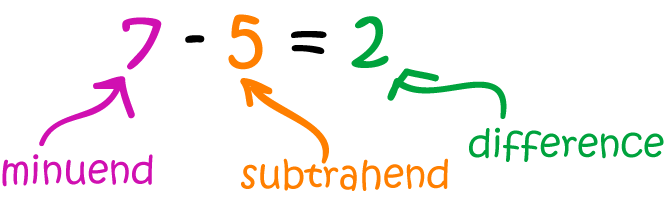minuend - subtrahend = difference.
Here,
• The minuend is the number that is subtracted from
• The subtrahend is the number that is taken away.
• The difference is the result of the subtraction.

Subtraction and addition are opposites of each other. So, subtraction provides a way to "undo" addition, and addition provides a way to "undo" subtraction.

When we know a subtraction fact, we can work out addition facts, and when we know addition facts, we can work out subtraction facts.

For example, we know that $7 - 4 = 3$. The related addition fact is $3 + 4 = 7$.

Let's try using this addition fact to work out some related subtraction facts: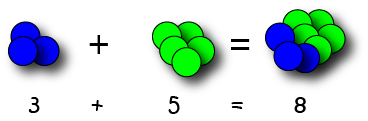If we add $2$ blue balls to $5$ red balls, we get $7$ balls altogether. So, $2 + 5 = 7$.

The related subtraction facts are:

• $7 - 2 = 5$
• $7 - 5 = 2$

When you need to do a subtraction, like $9 - 7$, you can ask yourself a related addition question: "What number do I add to $7$ to get $9$?". The answer is $2$, so $9 - 7 = 2$.

### Taking Bigger Numbers Away

One day, someone is going to ask you to take some bigger numbers than just $1$ or $2$ away. Don't panic, there are methods that you can learn to do this.

These include

• Subtraction with Regrouping (you might call it trading or borrowing). This is what most people do.
• Using number lines (or your ruler) to do the subtraction.

Oh, yes! By the way, $9 - 6 = 3$.

### Description

This mini book covers the core of Math for Foundation, Grade 1 and Grade 2 mathematics including

1. Numbers
3. Subtraction
4. Division
5. Algebra
6. Geometry
7. Data
8. Estimation
9. Probability/Chance
10. Measurement
11. Time
12. Money
13. and much more

This material is provided free of cost for Parent looking for some tricks for their Prekinder, Kinder, Prep, Year 1 and Year 2 children

### Learning Objectives

These lessons are for kids aged 4-8 with the core objective to expose their brains to concepts of addition, subtraction, division, algebra and much more.

Author: Subject Coach
You must be logged in as Student to ask a Question.